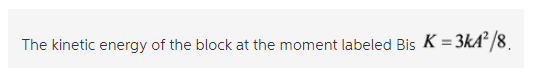# Find the kinetic energy K of the block at the moment labeled B

Find the kinetic energy K of the block at the moment labeled B. Express your answer in terms of
k and A.Concepts and reason
The concepts used to solve this problem are velocity of particle in simple harmonic motion and the kinetic energy.
Here the spring mass system is executing simple harmonic motion.
Use the expression for velocity of particle in simple harmonic motion to find the velocity of the block which attached to the spring
Then use the kinetic energy expression relating mass and velocity to find the kinetic energy of the block.

Fundamentals
In Simple harmonic motion the restoring force is directly proportional to the displacement of the object and the direction of force will be opposite to the displacement.
Expression for the speed of the block in simple harmonic motion is,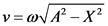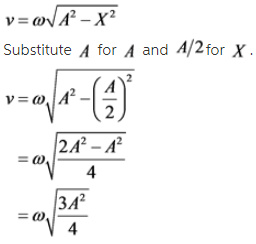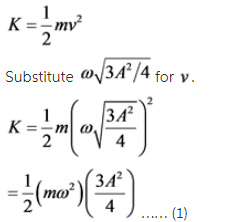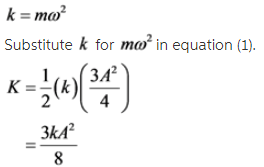Therefore, the kinetic energy of the block at the moment labeled B is